# Common Products and Factors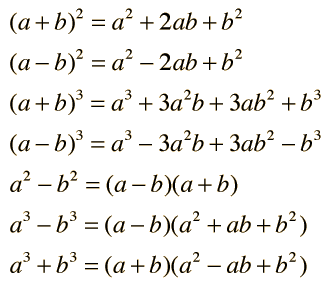### Any power of a binomial can be obtained from the Binomial Theorem.

Index

 HyperPhysics****HyperMath*****Algebra R Nave
Go Back

# Binomial Theorem

For any value of n, whether positive, negative, integer or non-integer, the value of the nth power of a binomial is given by: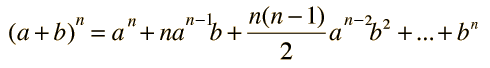There are many binomial expansion applications in physics.

Index

 HyperPhysics****HyperMath*****Algebra R Nave
Go Back

# Binomial Expansion

For any power of n, the binomial (a + x) can be expanded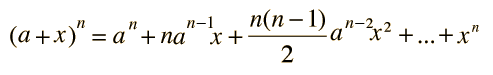This is particularly useful when x is very much less than a so that the first few terms provide a good approximation of the value of the expression. There will always be n+1 terms and the general form is: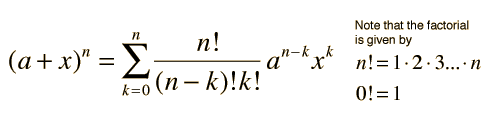** Examples

### Applications

Kinematic time dilation Gravitational time dilation
Relativity factor gamma ...
Index

 HyperPhysics****HyperMath*****Algebra R Nave
Go Back

# Binomial Expansion Examples

The binomial expansion is a useful example of a series. Some common examples of its application are: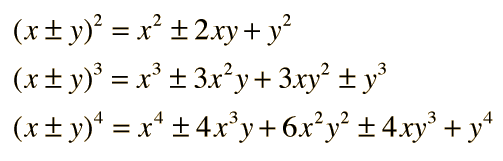Index

 HyperPhysics****HyperMath*****Algebra R Nave
Go Back